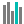#NEGBINOM.DIST (PQL - xl)

This function returns the negative binomial distribution

• Library: PQL \ Spreadsheet \ Statistical
• Compatibility: Any content (regardless of data source) in the Tabulate spreadsheet module

#### Syntax

NEGBINOM.DIST(number f, number s, probability s, cumulative)

##### Function Arguments
 Name Description Type Optional number f An integer representing the number of failures in trials Number number s An integer representing the threshold number of successes Number probability s Probability of success on each trial A number between 0 and 1 Number cumulative A logical value that determines the form of the function. If cumulative is TRUE, this function returns the cumulative distribution function; if FALSE, it returns the probability density function StringOrNumber